Elementary Algebra

# 9.6Solve Equations with Square Roots

Elementary Algebra9.6 Solve Equations with Square Roots

### Learning Objectives

By the end of this section, you will be able to:

• Use square roots in applications

### Be Prepared 9.6

Before you get started, take this readiness quiz.

1. Simplify: $99$ $9292$.
If you missed this problem, review Example 9.1 and Example 1.19.
2. Solve: $5(x+1)−4=3(2x−7)5(x+1)−4=3(2x−7)$.
If you missed this problem, review Example 2.42.
3. Solve: $n2−6n+8=0n2−6n+8=0$.
If you missed this problem, review Example 7.73.

In this section we will solve equations that have the variable in the radicand of a square root. Equations of this type are called radical equations.

An equation in which the variable is in the radicand of a square root is called a radical equation.

As usual, in solving these equations, what we do to one side of an equation we must do to the other side as well. Since squaring a quantity and taking a square root are ‘opposite’ operations, we will square both sides in order to remove the radical sign and solve for the variable inside.

But remember that when we write $aa$ we mean the principal square root. So $a≥0a≥0$ always. When we solve radical equations by squaring both sides we may get an algebraic solution that would make $aa$ negative. This algebraic solution would not be a solution to the original radical equation; it is an extraneous solution. We saw extraneous solutions when we solved rational equations, too.

### Example 9.74

For the equation $x+2=xx+2=x$:

Is $x=2x=2$ a solution? Is $x=−1x=−1$ a solution?

### Try It 9.147

For the equation $x+6=xx+6=x$:

Is $x=−2x=−2$ a solution? Is $x=3x=3$ a solution?

### Try It 9.148

For the equation $−x+2=x−x+2=x$:

Is $x=−2x=−2$ a solution? Is $x=1x=1$ a solution?

Now we will see how to solve a radical equation. Our strategy is based on the relation between taking a square root and squaring.

$Fora≥0,(a)2=aFora≥0,(a)2=a$

### Example 9.75

#### How to Solve Radical Equations

Solve: $2x−1=72x−1=7$.

### Try It 9.149

Solve: $3x−5=53x−5=5$.

### Try It 9.150

Solve: $4x+8=64x+8=6$.

### How To

1. Step 1. Isolate the radical on one side of the equation.
2. Step 2. Square both sides of the equation.
3. Step 3. Solve the new equation.
4. Step 4. Check the answer.

### Example 9.76

Solve: $5n−4−9=05n−4−9=0$.

### Try It 9.151

Solve: $3m+2−5=03m+2−5=0$.

### Try It 9.152

Solve: $10z+1−2=010z+1−2=0$.

### Example 9.77

Solve: $3y+5+2=53y+5+2=5$.

### Try It 9.153

Solve: $3p+3+3=53p+3+3=5$.

### Try It 9.154

Solve: $5q+1+4=65q+1+4=6$.

When we use a radical sign, we mean the principal or positive root. If an equation has a square root equal to a negative number, that equation will have no solution.

### Example 9.78

Solve: $9k−2+1=09k−2+1=0$.

### Try It 9.155

Solve: $2r−3+5=02r−3+5=0$.

### Try It 9.156

Solve: $7s−3+2=07s−3+2=0$.

If one side of the equation is a binomial, we use the binomial squares formula when we square it.

### Binomial Squares

$(a+b)2=a2+2ab+b2(a−b)2=a2−2ab+b2(a+b)2=a2+2ab+b2(a−b)2=a2−2ab+b2$

Don’t forget the middle term!

### Example 9.79

Solve: $p−1+1=pp−1+1=p$.

### Try It 9.157

Solve: $x−2+2=xx−2+2=x$.

### Try It 9.158

Solve: $y−5+5=yy−5+5=y$.

### Example 9.80

Solve: $r+4−r+2=0r+4−r+2=0$.

### Try It 9.159

Solve: $m+9−m+3=0m+9−m+3=0$.

### Try It 9.160

Solve: $n+1−n+1=0n+1−n+1=0$.

When there is a coefficient in front of the radical, we must square it, too.

### Example 9.81

Solve: $33x−5−8=433x−5−8=4$.

### Try It 9.161

Solve: $24a+2−16=1624a+2−16=16$.

### Try It 9.162

Solve: $36b+3−25=5036b+3−25=50$.

### Example 9.82

Solve: $4z−3=3z+24z−3=3z+2$.

### Try It 9.163

Solve: $2x−5=5x+32x−5=5x+3$.

### Try It 9.164

Solve: $7y+1=2y−57y+1=2y−5$.

Sometimes after squaring both sides of an equation, we still have a variable inside a radical. When that happens, we repeat Step 1 and Step 2 of our procedure. We isolate the radical and square both sides of the equation again.

### Example 9.83

Solve: $m+1=m+9m+1=m+9$.

### Try It 9.165

Solve: $x+3=x+5x+3=x+5$.

### Try It 9.166

Solve: $m+5=m+16m+5=m+16$.

### Example 9.84

Solve: $q−2+3=4q+1q−2+3=4q+1$.

### Try It 9.167

Solve: $y−3+2=4y+2y−3+2=4y+2$.

### Try It 9.168

Solve: $n−4+5=3n+3n−4+5=3n+3$.

### Use Square Roots in Applications

As you progress through your college courses, you’ll encounter formulas that include square roots in many disciplines. We have already used formulas to solve geometry applications.

We will use our Problem Solving Strategy for Geometry Applications, with slight modifications, to give us a plan for solving applications with formulas from any discipline.

### How To

#### Solve applications with formulas.

1. Step 1. Read the problem and make sure all the words and ideas are understood. When appropriate, draw a figure and label it with the given information.
2. Step 2. Identify what we are looking for.
3. Step 3. Name what we are looking for by choosing a variable to represent it.
4. Step 4. Translate into an equation by writing the appropriate formula or model for the situation. Substitute in the given information.
5. Step 5. Solve the equation using good algebra techniques.
6. Step 6. Check the answer in the problem and make sure it makes sense.
7. Step 7. Answer the question with a complete sentence.

We used the formula $A=L·WA=L·W$ to find the area of a rectangle with length L and width W. A square is a rectangle in which the length and width are equal. If we let s be the length of a side of a square, the area of the square is $s2s2$.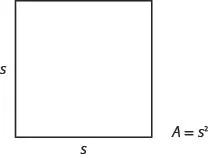The formula $A=s2A=s2$ gives us the area of a square if we know the length of a side. What if we want to find the length of a side for a given area? Then we need to solve the equation for s.

$A=s2 Take the square root of both sides.A=s2 Simplify.A=s A=s2 Take the square root of both sides.A=s2 Simplify.A=s$

We can use the formula $s=As=A$ to find the length of a side of a square for a given area.

### Area of a Square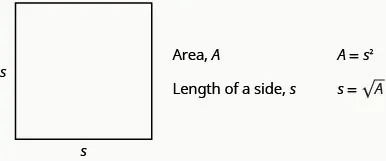We will show an example of this in the next example.

### Example 9.85

Mike and Lychelle want to make a square patio. They have enough concrete to pave an area of 200 square feet. Use the formula $s=As=A$ to find the length of each side of the patio. Round your answer to the nearest tenth of a foot.

### Try It 9.169

Katie wants to plant a square lawn in her front yard. She has enough sod to cover an area of 370 square feet. Use the formula $s=As=A$ to find the length of each side of her lawn. Round your answer to the nearest tenth of a foot.

### Try It 9.170

Sergio wants to make a square mosaic as an inlay for a table he is building. He has enough tile to cover an area of 2704 square centimeters. Use the formula $s=As=A$ to find the length of each side of his mosaic. Round your answer to the nearest tenth of a foot.

Another application of square roots has to do with gravity.

### Falling Objects

On Earth, if an object is dropped from a height of $hh$ feet, the time in seconds it will take to reach the ground is found by using the formula,

$t=h4t=h4$

For example, if an object is dropped from a height of 64 feet, we can find the time it takes to reach the ground by substituting $h=64h=64$ into the formula.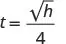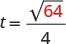Take the square root of 64.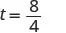Simplify the fraction.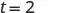It would take 2 seconds for an object dropped from a height of 64 feet to reach the ground.

### Example 9.86

Christy dropped her sunglasses from a bridge 400 feet above a river. Use the formula $t=h4t=h4$ to find how many seconds it took for the sunglasses to reach the river.

### Try It 9.171

A helicopter dropped a rescue package from a height of 1,296 feet. Use the formula $t=h4t=h4$ to find how many seconds it took for the package to reach the ground.

### Try It 9.172

A window washer dropped a squeegee from a platform 196 feet above the sidewalk Use the formula $t=h4t=h4$ to find how many seconds it took for the squeegee to reach the sidewalk.

Police officers investigating car accidents measure the length of the skid marks on the pavement. Then they use square roots to determine the speed, in miles per hour, a car was going before applying the brakes.

### Skid Marks and Speed of a Car

If the length of the skid marks is d feet, then the speed, s, of the car before the brakes were applied can be found by using the formula,

$s=24ds=24d$

### Example 9.87

After a car accident, the skid marks for one car measured 190 feet. Use the formula $s=24ds=24d$ to find the speed of the car before the brakes were applied. Round your answer to the nearest tenth.

### Try It 9.173

An accident investigator measured the skid marks of the car. The length of the skid marks was 76 feet. Use the formula $s=24ds=24d$ to find the speed of the car before the brakes were applied. Round your answer to the nearest tenth.

### Try It 9.174

The skid marks of a vehicle involved in an accident were 122 feet long. Use the formula $s=24ds=24d$ to find the speed of the vehicle before the brakes were applied. Round your answer to the nearest tenth.

### Section 9.6 Exercises

#### Practice Makes Perfect

In the following exercises, check whether the given values are solutions.

389.

For the equation $x+12=xx+12=x$:
Is $x=4x=4$ a solution?
Is $x=−3x=−3$ a solution?

390.

For the equation $−y+20=y−y+20=y$:
Is $y=4y=4$ a solution?
Is $y=−5y=−5$ a solution?

391.

For the equation $t+6=tt+6=t$:
Is $t=−2t=−2$ a solution?
Is $t=3t=3$ a solution?

392.

For the equation $u+42=uu+42=u$:
Is $u=−6u=−6$ a solution?
Is $u=7u=7$ a solution?

In the following exercises, solve.

393.

$5 y + 1 = 4 5 y + 1 = 4$

394.

$7 z + 15 = 6 7 z + 15 = 6$

395.

$5 x − 6 = 8 5 x − 6 = 8$

396.

$4 x − 3 = 7 4 x − 3 = 7$

397.

$2 m − 3 − 5 = 0 2 m − 3 − 5 = 0$

398.

$2 n − 1 − 3 = 0 2 n − 1 − 3 = 0$

399.

$6 v − 2 − 10 = 0 6 v − 2 − 10 = 0$

400.

$4 u + 2 − 6 = 0 4 u + 2 − 6 = 0$

401.

$5 q + 3 − 4 = 0 5 q + 3 − 4 = 0$

402.

$4 m + 2 + 2 = 6 4 m + 2 + 2 = 6$

403.

$6 n + 1 + 4 = 8 6 n + 1 + 4 = 8$

404.

$2 u − 3 + 2 = 0 2 u − 3 + 2 = 0$

405.

$5 v − 2 + 5 = 0 5 v − 2 + 5 = 0$

406.

$3 z − 5 + 2 = 0 3 z − 5 + 2 = 0$

407.

$2 m + 1 + 4 = 0 2 m + 1 + 4 = 0$

408.

$u−3+3=uu−3+3=u$
$x+1−x+1=0x+1−x+1=0$

409.

$v−10+10=vv−10+10=v$
$y+4−y+2=0y+4−y+2=0$

410.

$r−1−r=−1r−1−r=−1$
$z+100−z+10=0z+100−z+10=0$

411.

$s−8−s=−8s−8−s=−8$
$w+25−w+5=0w+25−w+5=0$

412.

$3 2 x − 3 − 20 = 7 3 2 x − 3 − 20 = 7$

413.

$2 5 x + 1 − 8 = 0 2 5 x + 1 − 8 = 0$

414.

$2 8 r + 1 − 8 = 2 2 8 r + 1 − 8 = 2$

415.

$3 7 y + 1 − 10 = 8 3 7 y + 1 − 10 = 8$

416.

$3 u − 2 = 5 u + 1 3 u − 2 = 5 u + 1$

417.

$4 v + 3 = v − 6 4 v + 3 = v − 6$

418.

$8 + 2 r = 3 r + 10 8 + 2 r = 3 r + 10$

419.

$12 c + 6 = 10 − 4 c 12 c + 6 = 10 − 4 c$

420.

$a+2=a+4a+2=a+4$
$b−2+1=3b+2b−2+1=3b+2$

421.

$r+6=r+8r+6=r+8$
$s−3+2=s+4s−3+2=s+4$

422.

$u+1=u+4u+1=u+4$
$n−5+4=3n+7n−5+4=3n+7$

423.

$x+10=x+2x+10=x+2$
$y−2+2=2y+4y−2+2=2y+4$

424.

$2 y + 4 + 6 = 0 2 y + 4 + 6 = 0$

425.

$8 u + 1 + 9 = 0 8 u + 1 + 9 = 0$

426.

$a + 1 = a + 5 a + 1 = a + 5$

427.

$d − 2 = d − 20 d − 2 = d − 20$

428.

$6 s + 4 = 8 s − 28 6 s + 4 = 8 s − 28$

429.

$9 p + 9 = 10 p − 6 9 p + 9 = 10 p − 6$

Use Square Roots in Applications

In the following exercises, solve. Round approximations to one decimal place.

430.

Landscaping Reed wants to have a square garden plot in his backyard. He has enough compost to cover an area of 75 square feet. Use the formula $s=As=A$ to find the length of each side of his garden. Round your answer to the nearest tenth of a foot.

431.

Landscaping Vince wants to make a square patio in his yard. He has enough concrete to pave an area of 130 square feet. Use the formula $s=As=A$ to find the length of each side of his patio. Round your answer to the nearest tenth of a foot.

432.

Gravity While putting up holiday decorations, Renee dropped a light bulb from the top of a 64 foot tall tree. Use the formula $t=h4t=h4$ to find how many seconds it took for the light bulb to reach the ground.

433.

Gravity An airplane dropped a flare from a height of 1024 feet above a lake. Use the formula $t=h4t=h4$ to find how many seconds it took for the flare to reach the water.

434.

Gravity A hang glider dropped his cell phone from a height of 350 feet. Use the formula $t=h4t=h4$ to find how many seconds it took for the cell phone to reach the ground.

435.

Gravity A construction worker dropped a hammer while building the Grand Canyon skywalk, 4000 feet above the Colorado River. Use the formula $t=h4t=h4$ to find how many seconds it took for the hammer to reach the river.

436.

Accident investigation The skid marks for a car involved in an accident measured 54 feet. Use the formula $s=24ds=24d$ to find the speed of the car before the brakes were applied. Round your answer to the nearest tenth.

437.

Accident investigation The skid marks for a car involved in an accident measured 216 feet. Use the formula $s=24ds=24d$ to find the speed of the car before the brakes were applied. Round your answer to the nearest tenth.

438.

Accident investigation An accident investigator measured the skid marks of one of the vehicles involved in an accident. The length of the skid marks was 175 feet. Use the formula $s=24ds=24d$ to find the speed of the vehicle before the brakes were applied. Round your answer to the nearest tenth.

439.

Accident investigation An accident investigator measured the skid marks of one of the vehicles involved in an accident. The length of the skid marks was 117 feet. Use the formula $s=24ds=24d$ to find the speed of the vehicle before the brakes were applied. Round your answer to the nearest tenth.

#### Writing Exercises

440.

Explain why an equation of the form $x+1=0 x+1=0$ has no solution.

441.
1. Solve the equation $r+4−r+2=0r+4−r+2=0$.
2. Explain why one of the “solutions” that was found was not actually a solution to the equation.

#### Self Check

After completing the exercises, use this checklist to evaluate your mastery of the objectives of this section.After reviewing this checklist, what will you do to become confident for all objectives?

Order a print copy

As an Amazon Associate we earn from qualifying purchases.